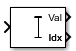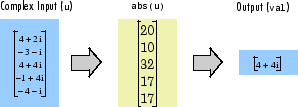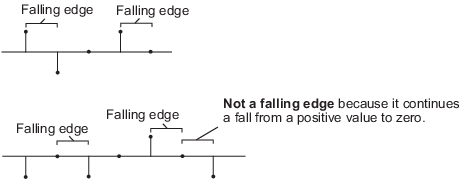# Maximum

Maximum values of input or sequence of inputs

•Libraries:
DSP System Toolbox / Statistics
DSP System Toolbox HDL Support / Statistics

## Description

The Maximum block identifies the value and position of the largest element in each row or column of the input, or along vectors of a specified dimension of the input. It can also compute the maximum value of the entire input. The Maximum block can also track the maximum values in a sequence of inputs over a period of time. The Mode parameter specifies the block's mode of operation and can be set to one of the following:

• `Value` — The block outputs the maximum values in the specified dimension.

• `Index` — The block outputs the index array of the maximum values in the specified dimension.

• `Value and Index` — The block outputs the maximum values and the corresponding index array in the specified dimension.

• `Running` — The block tracks the maximum values in a sequence of inputs over a period of time.

You can specify the dimension using the Find the maximum value over parameter.

Note

The Running mode in the Maximum block will be removed in a future release. To compute the running maximum in Simulink®, use the Moving Maximum block instead.

## Ports

### Input

expand all

The block accepts real-valued or complex-valued multichannel and multidimensional inputs. The input can be floating-point, fixed-point, or Boolean. Real fixed-point inputs can be either signed or unsigned. Complex fixed-point inputs must be signed.

This port is unnamed until you set the Mode parameter to `Running` and the Reset port parameter to any option other than `None`.

Data Types: `single` | `double` | `int8` | `int16` | `int32` | `int64` | `uint8` | `uint16` | `uint32` | `uint64` | `Boolean` | `fixed point`
Complex Number Support: Yes

Specify the reset event that causes the block to reset the running maximum. The sample time of the Rst input must be a positive integer multiple of the input sample time.

#### Dependencies

To enable this port, set the Mode parameter to `Running` and the Reset port parameter to any option other than `None`.

Data Types: `single` | `double` | `int8` | `int16` | `int32` | `int64` | `uint8` | `uint16` | `uint32` | `Boolean`

### Output

expand all

The data type of the maximum value matches the data type of the input.

When the Mode parameter is set to either `Value and Index` or `Value`, the following applies:

• The size of the dimension for which the block computes the maximum value is 1. The sizes of all other dimensions match those of the input array. For example, when the input is an M-by-N-by-P array, with the dimension set to `1`, the block outputs a 1-by-N-by-P array. When the dimension is set to `3`, the block outputs a two-dimensional M-by-N matrix.

• When the input is an M-by-N matrix, with the dimension set to `1`, the block outputs a 1-by-N matrix.

If you specify the block to compute the maximum value over the entire input, the block outputs a scalar.

When the Mode parameter is set `Running`, the block tracks the maximum value of each channel in a time sequence of M-by-N inputs. In this mode, you must also specify the Input processing parameter as one of the following:

• ```Elements as channels (sample based)``` — The block treats each element of the input as a separate channel. For a three-dimensional input signal of size M-by-N-by-P, the block outputs an M-by-N-by-P array. Each yijk element of the output contains the maximum value observed in element uijk for all inputs since the last reset.

When a reset event occurs, the running maximum yijk in the current frame is reset to the element uijk.

• ```Columns as channels (frame based)``` — The block treats each column of the input as a separate channel. This option does not support an N-dimensional input signal, where N > 2. For a two-dimensional input signal of size M-by-N, the block outputs an M-by-N matrix. Each element yij of the output contains the maximum value observed in the jth column of all inputs since the last reset, up to and including element uij of the current input.

When a reset event occurs, the running maximum for each channel becomes the maximum value of all the samples in the current input frame, up to and including the current input sample.

The block resets the running maximum whenever a reset event is detected at the optional Rst port. The reset sample time must be a positive integer multiple of the input sample time.

#### Dependencies

To enable this port, set the Mode parameter to either `Value and Index` or `Value`.

Data Types: `single` | `double` | `int8` | `int16` | `int32` | `int64` | `uint8` | `uint16` | `uint32` | `uint64` | `Boolean` | `fixed point`
Complex Number Support: Yes

When the input is `double`, the index values are also `double`. Otherwise, the index values are `uint32`.

#### Dependencies

To enable this port, set the Mode parameter to either `Value and Index` or `Index`.

Data Types: `double` | `uint32`

## Parameters

expand all

### Main Tab

When the Mode parameter is set to:

• `Value` — The block computes the maximum value in each row or column of the input, or along vectors of a specified dimension of the input. It can also compute the maximum value of the entire input at each sample time, and outputs the array, y. Each element in the output is the maximum value in the corresponding column, row, vector, or entire input. The output y depends on the setting of the Find the maximum value over parameter. Consider a three dimensional input signal of size M-by-N-by-P. Set Find the maximum value over to:

• `Each row` — The output y at each sample time consists of an M-by-1-by-P array, where each element contains the maximum value of each vector over the second dimension of the input. For an M-by-N matrix input, the output at each sample time is an M-by-1 column vector.

• `Each column` — The output y at each sample time consists of a 1-by-N-by-P array, where each element contains the maximum value of each vector over the first dimension of the input. For an M-by-N matrix input, the output at each sample time is a 1-by-N row vector.

In this mode, the block treats length-M unoriented vector inputs as M-by-1 column vectors.

• `Entire input` — The output y at each sample time is a scalar that contains the maximum value in the M-by-N-by-P input matrix.

• `Specified dimension` — The output y at each sample time depends on Dimension. If Dimension is set to `1`, the output is the same as when you select ```Each column```. If Dimension is set to `2`, the output is the same as when you select ```Each row```. If Dimension is set to `3`, the output at each sample time is an M-by-N matrix containing the maximum value of each vector over the third dimension of the input.

Complex Inputs

For complex inputs, the block selects the value in each row or column of the input, along vectors of a specified dimension of the input, or of the entire input that has the maximum magnitude squared as shown in the following figure. For complex value $u=a+bi$, the magnitude squared is ${a}^{2}+{b}^{2}$.• `Index` — The block computes the maximum value in each row or column of the input, along vectors of a specified dimension of the input, or of the entire input, and outputs the index array I. Each element in I is an integer indexing the maximum value in the corresponding column, row, vector, or entire input. The output I depends on the setting of the Find the maximum value over parameter. Consider a three-dimensional input signal of size M-by-N-by-P:

• `Each row` — The output I at each sample time consists of an M-by-1-by-P array, where each element contains the index of the maximum value of each vector over the second dimension of the input. For an input that is an M-by-N matrix, the output at each sample time is an M-by-1 column vector.

• `Each column` — The output I at each sample time consists of a 1-by-N-by-P array, where each element contains the index of the maximum value of each vector over the first dimension of the input. For an input that is an M-by-N matrix, the output at each sample time is a 1-by-N row vector.

In this mode, the block treats length-M unoriented vector inputs as M-by-1 column vectors.

• `Entire input` — The output I at each sample time is a 1-by-3 vector that contains the location of the maximum value in the M-by-N-by-P input matrix. For an input that is an M-by-N matrix, the output is a 1-by-2 vector.

• `Specified dimension` — The output I at each sample time depends on Dimension. If Dimension is set to 1, the output is the same as when you select `Each column`. If Dimension is set to 2, the output is the same as when you select `Each row`. If Dimension is set to 3, the output at each sample time is an M-by-N matrix containing the indices of the maximum values of each vector over the third dimension of the input.

When a maximum value occurs more than once, the computed index corresponds to the first occurrence. For example, when the input is the column vector ```[3 2 1 2 3]'```, the computed one-based index of the maximum value is `1`, rather than `5` when ```Each column``` is selected.

• `Value and Index` — The block outputs the maximum value in each row or column of the input, along vectors of a specified dimension of the input, or of the entire input, and the corresponding index array I.

• `Running` — The block tracks the maximum value of each channel in a time sequence of M-by-N inputs. In this mode, you must also specify the Input processing parameter as one of the following:

• ```Elements as channels (sample based)``` — The block treats each element of the input as a separate channel. For a three-dimensional input signal of size M-by-N-by-P, the block outputs an M-by-N-by-P array. Each yijk element of the output contains the maximum value observed in element uijk for all inputs since the last reset.

When a reset event occurs, the running maximum yijk in the current frame is reset to the element uijk.

• ```Columns as channels (frame based)``` — The block treats each column of the input as a separate channel. This option does not support an N-dimensional input signal, where N > 2. For a two-dimensional input signal of size M-by-N, the block outputs an M-by-N matrix. Each element yij of the output contains the maximum value observed in the jth column of all inputs since the last reset, up to and including element uij of the current input.

When a reset event occurs, the running maximum for each channel becomes the maximum value of all the samples in the current input frame, up to and including the current input sample.

The block resets the running maximum whenever a reset event is detected at the optional Rst port. The reset sample time must be a positive integer multiple of the input sample time.

Running Mode for Variable-Size Inputs

When the input is a variable-size signal, and you set the Mode to `Running`, then:

• If you set the Input processing parameter to ```Elements as channels (sample based)```, the state is reset.

• If you set the Input processing parameter to ```Columns as channels (frame based)```, then:

• When the input size difference is in the number of channels (columns), the state is reset.

• When the input size difference is in the length of channels (rows), there is no reset and the running operation is carried out as usual.

Specify whether the index of the maximum value is reported using one-based or zero-based numbering.

#### Dependencies

To enable this parameter, set Mode to either `Index` or ```Value and Index```.

• `Each column` — The block outputs the maximum value over each column.

• `Each row` — The block outputs the maximum value over each row.

• `Entire input` — The block outputs the maximum value over the entire input.

• `Specified dimension` — The block outputs the maximum value over the dimension, specified in the Dimension parameter.

#### Dependencies

To enable this parameter, set Mode to `Value and Index`, `Value`, or `Index`.

Specify the dimension (one-based value) of the input signal over which the block computes the maximum. The value of this parameter must be greater than 0 and less than the number of dimensions in the input signal.

#### Dependencies

To enable this parameter, set Find the maximum value over to ```Specified dimension```.

• `Columns as channels (frame based)` — The block treats each column of the input as a separate channel. This option does not support an N-dimensional input signal, where N > 2. For a two-dimensional input signal of size M-by-N, the block outputs an M-by-N matrix. Each element yij of the output contains the maximum value observed in the jth column of all inputs since the last reset, up to and including element uij of the current input.

When a reset event occurs, the running maximum for each channel becomes the maximum value of all the samples in the current input frame, up to and including the current input sample.

• `Elements as channels (sample based)` — The block treats each element of the input as a separate channel. For a three-dimensional input signal of size M-by-N-by-P, the block outputs an M-by-N-by-P array. Each yijk element of the output contains the maximum value observed in element uijk for all inputs since the last reset.

When a reset event occurs, the running maximum yijk in the current frame is reset to the element uijk.

#### Dependencies

To enable this parameter, set Mode to `Running`.

The block resets the running maximum whenever a reset event is detected at the optional Rst port. The reset sample time must be a positive integer, which is a multiple of the input sample time.

• `None` — Disables the Rst port.

• `Rising edge` — Triggers a reset operation when the Rst input does one of the following:

• Rises from a negative value to a positive value or zero.

• Rises from zero to a positive value, where the rise is not a continuation of a rise from a negative value to zero.• `Falling edge` — Triggers a reset operation when the Rst input does one of the following:

• Falls from a positive value to a negative value or zero.

• Falls from zero to a negative value, where the fall is not a continuation of a fall from a positive value to zero.• `Either edge` — Triggers a reset operation when the Rst input is a `Rising edge` or `Falling edge`.

• `Non-zero sample` — Triggers a reset operation at each sample time that the Rst input is not zero.

Note

When running simulations in the Simulink `MultiTasking` mode, reset signals have a one-sample latency. Therefore, when the block detects a reset event, there is a one-sample delay at the reset port rate before the block applies the reset. For more information on latency and the Simulink tasking modes, see Excess Algorithmic Delay (Tasking Latency) and Time-Based Scheduling and Code Generation (Simulink Coder).

#### Dependencies

To enable this parameter, set Mode to `Running`.

### Data Types Tab

Note

To use these parameters, the data input must be complex and fixed-point.

Specify the rounding mode for fixed-point operations as one of the following:

• `Floor`

• `Ceiling`

• `Convergent`

• `Nearest`

• `Round`

• `Simplest`

• `Zero`

For more details, see rounding mode.

When you select this parameter, the block saturates the result of its fixed-point operation. When you clear this parameter, the block wraps the result of its fixed-point operation. For details on `saturate` and `wrap`, see overflow mode for fixed-point operations.

Product output specifies the data type of the output of a product operation in the Maximum block. For more information on the product output data type, see Multiplication Data Types.

• `Inherit: Same as input` — The block specifies the product output data type to be the same as the input data type.

• `fixdt([],16,0)` — The block specifies an autosigned, binary-point, scaled, fixed-point data type with a word length of 16 bits and a fraction length of 0.

Alternatively, you can set the Product output data type by using the Data Type Assistant. To use the assistant, click the button.

For more information on the data type assistant, see Specify Data Types Using Data Type Assistant (Simulink).

Accumulator specifies the data type of the output of an accumulation operation in the Maximum block.

• `Inherit: Same as product output` — The block specifies the accumulator data type to be the same as the product output data type.

• `Inherit: Same as input` — The block specifies the accumulator data type to be the same as the input data type.

• `fixdt([],16,0)` — The block specifies an autosigned, binary-point, scaled, fixed-point data type with a word length of 16 bits and a fraction length of 0.

Alternatively, you can set the Accumulator data type by using the Data Type Assistant. To use the assistant, click the button.

For more information on the data type assistant, see Specify Data Types Using Data Type Assistant (Simulink).

Select this parameter to prevent the fixed-point tools from overriding the data types you specify in the block dialog box.

## Block Characteristics

 Data Types `double` | `fixed point` | `integer` | `single` Direct Feedthrough `no` Multidimensional Signals `no` Variable-Size Signals `yes` Zero-Crossing Detection `no`

expand all

## Version History

Introduced before R2006a